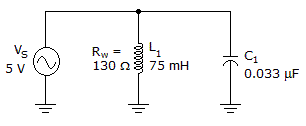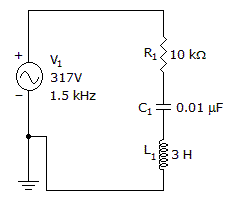# Electronics - RLC Circuits and Resonance

### Exercise :: RLC Circuits and Resonance - General Questions

11.What is the resonant frequency in the given circuit?

 A. 11.6 Hz B. 275.8 Hz C. 1.5 kHz D. 3.2 kHz

Answer: Option D

Explanation:

No answer description available for this question. Let us discuss.

12.

Series RLC voltage or impedance totals must be calculated by:

 A. subtracting the values B. graphing the angles C. adding vectors D. multiplying the values

Answer: Option C

Explanation:

No answer description available for this question. Let us discuss.

13.

What is the total current?A. 15.6 mA B. 17.8 mA C. 21.9 mA D. 26.0 mA

Answer: Option A

Explanation:

No answer description available for this question. Let us discuss.

14.

When XC = XL the circuit:

 A. draws maximum current B. applied voltage is zero C. is at resonance D. draws minimum current

Answer: Option C

Explanation:

No answer description available for this question. Let us discuss.

15.

How much current will flow in a series RLC circuit when VT = 100 V, XL = 160, XC = 80, and R = 60?

 A. 1 A B. 1 mA C. 6.28 A D. 10 A

Answer: Option A

Explanation:

No answer description available for this question. Let us discuss.

#### Current Affairs 2021

Interview Questions and Answers